﻿

### On semidirect product of semigroups

#### Abstract

Let X be a subset of a semigroup S. We denote by E(X) the set of idempotent elements Of X.An element a of a semigroup S is called E-inverse if there exists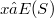$x ∈ E(S)$ such that$ax ∈ E(S)$. We note that the definition is not one-sided. Indeed, an a element of a semigroup S is E-inversive if there exists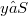$y ∈ S$ such that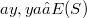$ay, ya ∈ E(S)$ (see , [l] p. 98). A semigroup S is called E-inversive if all its elements are E-inversive. This class of semigroups is extensive. All semigroups with a zero and all eventually regular semigroups  are E-inversive semigroups.Recently E-inversive semigroups reappeared in a paper by Hall and Munn  and in a paper by Mitsch . The special case of E-inversive semigroups with pairwise commuting idempotents, called E-dense, was considered by Margolis and Pin . Let S and T be semigroups, and let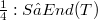$𝛼 : S → End(T)$ be a homomorphism of S into the endomorphism semigroup of T. If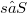$s ∈ S$ and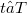$t ∈ T$, denote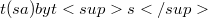$t(sa) by ts$. Thus, if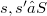$s,s' ∈ S$ and$t ∈ T$ then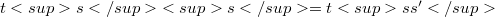${ts}s = tss'$. The semidirect product of S and T ,in that order, with strutture map (Y, consists of the set S x T equipped with the product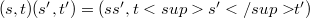$(s,t)(s',t') = (ss', {ts'}{t'})$. This product will be denoted by S _{𝛼}T. In this note we determine which semidirect products of semigroups are E-inversive semigroups and E-dense semigroups, respectively. It turns out that the case in which S induces only automorphism on T allows a particularly simple description. In , Preston has answered the analous question for regular semigroups and for inverse semigroups. For the terminology and for the definitions of the algebraic theory of semigroups, we refer to .

DOI Code: 10.1285/i15900932v9n2p189

Full Text: PDF